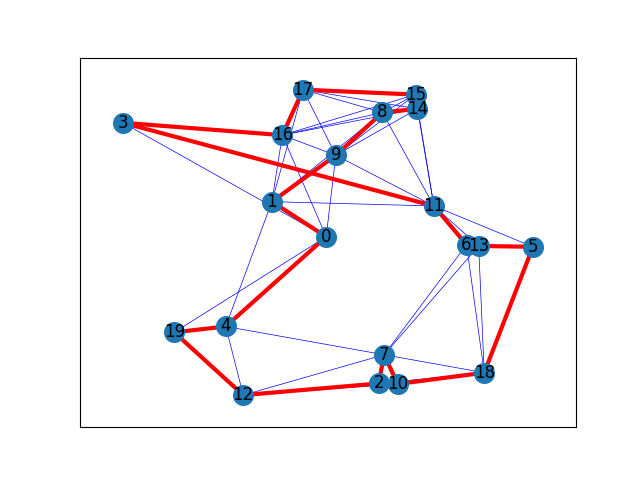# Traveling Salesman Problem#

This is an example of a drawing solution of the traveling salesman problem

The function is used to produce the solution is christofides, where given a set of nodes, it calculates the route of the nodes that the traveler has to follow in order to minimize the total cost.```The route of the traveller is: [0, 4, 19, 12, 2, 7, 10, 18, 5, 13, 6, 11, 3, 16, 17, 15, 14, 8, 9, 1, 0]
```

```import matplotlib.pyplot as plt
import networkx as nx
import networkx.algorithms.approximation as nx_app
import math

pos = nx.get_node_attributes(G, "pos")

# Depot should be at (0,0)
pos = (0.5, 0.5)

H = G.copy()

# Calculating the distances between the nodes as edge's weight.
for i in range(len(pos)):
for j in range(i + 1, len(pos)):
dist = math.hypot(pos[i] - pos[j], pos[i] - pos[j])
dist = dist

cycle = nx_app.christofides(G, weight="weight")
edge_list = list(nx.utils.pairwise(cycle))

# Draw closest edges on each node only
nx.draw_networkx_edges(H, pos, edge_color="blue", width=0.5)

# Draw the route
nx.draw_networkx(
G,
pos,
with_labels=True,
edgelist=edge_list,
edge_color="red",
node_size=200,
width=3,
)

print("The route of the traveller is:", cycle)
plt.show()
```

Total running time of the script: ( 0 minutes 0.094 seconds)

Gallery generated by Sphinx-Gallery